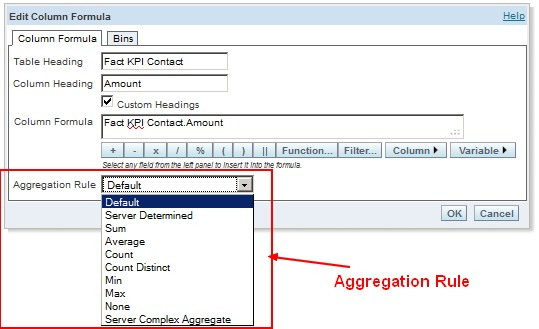# Function - (Aggregate | Aggregation)

Aggregate functions return a single value

from values that are in a aggregation relationship (ie a set)

This values are also known as summary because they try to summarize / describe a set of data

## List

### Computed

You compute generally over additive numeric data grouped by class attribute

### Selection

• FIRST() - Returns the first value
• LAST() - Returns the last value
• MAX() - Returns the largest value
• MIN() - Returns the smallest value
• Quantile - (Median|Middle) - Returns the median

## Implementation

### Mutative operation

Mutative accumulation for a sum

``````int sum = 0;
for (int x : numbers) {
sum += x;
}```
```

### Reduction operation

They are implemented as reduction operation

### Partition

Some operations like AVG are not partitionable. The computation can't therefore happens in parallel.

It is not valid to compute them on partitioned data because they are not commutative and associative.

You can still compute partial aggregates by transforming the non-commutative and associative function by commutative and associative function and, then roll them up.

Example: if:

• you want to compute AVG(x)
• you expand to SUM(x) / COUNT(x),
• You compute partition SUM(x) and COUNT(X) on each partition
• You sum them using SUM.

Recommended Pages(Data Processing|Data Integration)

Data processing is a more general term for manipulating data whereas data integration is the integration of data between two systems. Data Integration has roughly two data processing model: Model...(Function | Operator | Map | Mapping | Transformation | Method | Rule | Task | Subroutine)

Section computable function. A function is a callable unit that may be called: a procedure, a subrontine a routine, a method (belong to an objectmacrocomputablalgorithreusable blocargumentdevelopment...Dimensional Data Modeling - Descriptif Attribute (Dimensional Attribute)

A descriptif attribute is class attribute that describe a property or characteristic of a dimension. They are used to label, filter and/or group on. measures Typical attributes for a product dimension...Dimensional Data Modeling - Measure

In a dimensional model, a measure is a quantitative attribute of a fact (in a fact table) that is not a foreign key that creates a relationship to a dimension. A measure permits to quantify. A calculated...Function - Maximum (Max)

A page the max aggregate functionFunction - User Defined Functions (UDF)

User-defined_functionUser-defined Functions (UDFs) is a function provided by a developer and behave as a built-in function. It gives to the developer the possibility to enhance the function possibilities...Logical Data Modeling - Aggregate Data

Aggregate data are data that are in a aggregation relation (ie elements that are in a collection) An aggregate function is a function that is applied to aggregate data (ie A sum is applied to a collection...
Logical Data Modeling - Aggregation (Collection)

aggregation is a containment relationship where one or more entities are part of a container entity. In other word, aggregation is combining multiple pieces of data into one unit. The container entity...Number - Addition (or Sum, Sigma) or Total

The addition of all numbers in a set is called a total. Sum can be use as: an aggregate or a analytic function. It can follow the full syntax of the analytic function and in this way create It's...OBIEE - 10G/11G - OBIPS - Aggregation Rule

Aggregation rule. The aggregation rules apply to all aggregate function used in SQL statements : The 'aggregation rule' setting in Answer is applied to sub-totals and grand totals within the Answers...